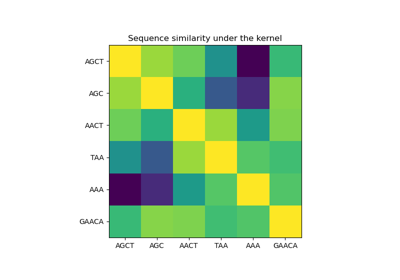# sklearn.gaussian_process.kernels.Kernel¶

class sklearn.gaussian_process.kernels.Kernel[source]

Base class for all kernels.

New in version 0.18.

Attributes
bounds

Returns the log-transformed bounds on the theta.

hyperparameters

Returns a list of all hyperparameter specifications.

n_dims

Returns the number of non-fixed hyperparameters of the kernel.

requires_vector_input

Returns whether the kernel is defined on fixed-length feature vectors or generic objects.

theta

Returns the (flattened, log-transformed) non-fixed hyperparameters.

Methods

 __call__(self, X[, Y, eval_gradient]) Evaluate the kernel. clone_with_theta(self, theta) Returns a clone of self with given hyperparameters theta. diag(self, X) Returns the diagonal of the kernel k(X, X). get_params(self[, deep]) Get parameters of this kernel. is_stationary(self) Returns whether the kernel is stationary. set_params(self, \*\*params) Set the parameters of this kernel.
__init__(self, /, *args, **kwargs)

Initialize self. See help(type(self)) for accurate signature.

abstract __call__(self, X, Y=None, eval_gradient=False)[source]

Evaluate the kernel.

property bounds

Returns the log-transformed bounds on the theta.

Returns
boundsndarray of shape (n_dims, 2)

The log-transformed bounds on the kernel’s hyperparameters theta

clone_with_theta(self, theta)[source]

Returns a clone of self with given hyperparameters theta.

Parameters
thetandarray of shape (n_dims,)

The hyperparameters

abstract diag(self, X)[source]

Returns the diagonal of the kernel k(X, X).

The result of this method is identical to np.diag(self(X)); however, it can be evaluated more efficiently since only the diagonal is evaluated.

Parameters
Xarray-like of shape (n_samples,)

Left argument of the returned kernel k(X, Y)

Returns
K_diagndarray of shape (n_samples_X,)

Diagonal of kernel k(X, X)

get_params(self, deep=True)[source]

Get parameters of this kernel.

Parameters
deepbool, default=True

If True, will return the parameters for this estimator and contained subobjects that are estimators.

Returns
paramsdict

Parameter names mapped to their values.

property hyperparameters

Returns a list of all hyperparameter specifications.

abstract is_stationary(self)[source]

Returns whether the kernel is stationary.

property n_dims

Returns the number of non-fixed hyperparameters of the kernel.

property requires_vector_input

Returns whether the kernel is defined on fixed-length feature vectors or generic objects. Defaults to True for backward compatibility.

set_params(self, **params)[source]

Set the parameters of this kernel.

The method works on simple kernels as well as on nested kernels. The latter have parameters of the form <component>__<parameter> so that it’s possible to update each component of a nested object.

Returns
self
property theta

Returns the (flattened, log-transformed) non-fixed hyperparameters.

Note that theta are typically the log-transformed values of the kernel’s hyperparameters as this representation of the search space is more amenable for hyperparameter search, as hyperparameters like length-scales naturally live on a log-scale.

Returns
thetandarray of shape (n_dims,)

The non-fixed, log-transformed hyperparameters of the kernel

## Examples using sklearn.gaussian_process.kernels.Kernel¶